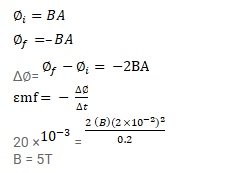# A conducting square loop having edges of length

Question:

A conducting square loop having edges of length $2.0 \mathrm{~cm}$ is rotated through $180^{\circ}$ about a diagonal in $0.20 \mathrm{~s}$. A magnetic field B exists in the region which is perpendicular to the loop in its initial position. If the average induced emf during the rotation is $20 \mathrm{mv}$, find the magnitude of the magnetic field.

Solution: